How Cheenta works to ensure student success?
Explore the Back-Story

# TOMATO Objective 44Try this problem from Test of Mathematics, TOMATO Objective problem number 44, useful for ISI B.Stat and B.Math.

Problem: TOMATO Objective 44

Suppose that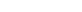(n> 2) are real numbers such that x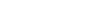for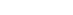. Consider the sum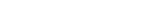where the summations are taken over all i, j, k: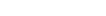and i, j, k are all distinct. Then S equals:

(A); (B) (n-3)(n-4); (C) (n-3)(n-4)(n-5); (D) none of the foregoing expressions;

Discussion: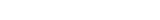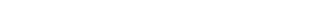Since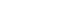Hence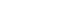Since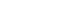and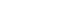Therefore.

Hence option D

Our ISI CMI Program

How to use invariance in Combinatorics – ISI Entrance Problem – Video

Try this problem from Test of Mathematics, TOMATO Objective problem number 44, useful for ISI B.Stat and B.Math.

Problem: TOMATO Objective 44

Suppose that(n> 2) are real numbers such that xfor. Consider the sumwhere the summations are taken over all i, j, k:and i, j, k are all distinct. Then S equals:

(A); (B) (n-3)(n-4); (C) (n-3)(n-4)(n-5); (D) none of the foregoing expressions;

Discussion:SinceHenceSinceandTherefore.

Hence option D

Our ISI CMI Program

How to use invariance in Combinatorics – ISI Entrance Problem – Video

This site uses Akismet to reduce spam. Learn how your comment data is processed.

### Knowledge Partner